NCERT Solutions for Class 8th: Ch 3 Understanding Quadrilaterals Maths Part-I

NCERT Solutions for Class 8th: Ch 3 Understanding Quadrilaterals Maths Part-I

Page No: 41

Exercise 3.1

1. Given here are some figures.Classify each of them on the basis of the following.
(a) Simple curve       (b) Simple closed curve       (c) Polygon
(d) Convex polygon         (e) Concave polygon

(a) Simple curve: 1, 2, 5, 6 and 7
(b) Simple closed curve: 1, 2, 5, 6 and 7
(c) Polygon: 1 and 2
(d) Convex polygon: 2
(e) Concave polygon: 1

2. How many diagonals does each of the following have?
(a) A convex quadrilateral         (b) A regular hexagon        (c) A triangle

(a) A convex quadrilateral: It has 2 diagonals.(b) A regular hexagon(c) A triangle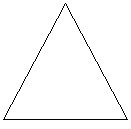3. What is the sum of the measures of the angles of a convex quadrilateral? Will this property hold if the quadrilateral is not convex? (Make a non-convex quadrilateral and try!)Let ABCD be a convex quadrilateral. We observe that the quadrilateral ABCD formed by two triangles i.e. ΔADC and ΔABC.
Since, we know that sum of interior angles of triangle is 180°. Thus, the sum of the measures of the angles is 180° 180° = 360°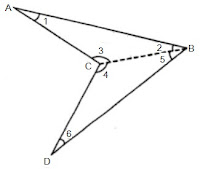Let us take another quadrilateral ABCD which is not convex and join BC which divides it into two triangles ΔABC and ΔBCD.
In ΔABC,
∠1 ∠2 ∠3 = 180°   (angle sum property of triangle)
In ΔBCD,
∠4 + ∠5 + ∠6 = 180°   (angle sum property of triangle)
Therefore,  ∠1 + ∠2 + ∠3 + ∠4 + ∠5 + ∠6 = 180° + 180°
⇒ ∠1 + ∠2 + ∠3 + ∠4 + ∠5 + ∠6 = 360°
⇒ ∠A + ∠B + ∠C + ∠D = 360°
Thus, this property hold if the quadrilateral is not convex.

4. Examine the table. (Each figure is divided into triangles and the sum of the angles deduced from that.)What can you say about the angle sum of a convex polygon with number of sides?
(a) 7            (b) 8            (c) 10             (d) n

The angle sum of a polygon having side n = (n-2)×180°
(a) 7
Here, n = 7
Thus, angle sum = (7-2)×180° = 5×180° = 900°

(b) 8
Here, n = 8
Thus, angle sum = (8-2)×180° = 6×180° = 1080°

(c) 10
Here, n = 10
Thus, angle sum = (10-2)×180° = 8×180° = 1440°

(d) n
Here, n = n
Thus, angle sum = (n-2)×180°

Page No: 42

5. What is a regular polygon?
State the name of a regular polygon of
(i) 3 sides          (ii) 4 sides           (iii) 6 sides

A polygon having sides of equal length and angles of equal measures is called regular polygon.
(i) A regular polygon of 3 sides is equilateral triangle.
(ii) A regular polygon of 4 sides is square.
(iii) A regular polygon of 6 sides is regular hexagon.

6. Find the angle measure x in the following figures.(a) The figure is having 4 sides. Hence, it is a quadrilateral.
Sum of angles of the quadrilateral = 360°
⇒ 50° + 130° + 120° + x = 360°
⇒ 300° + x = 360°
⇒ x = 360° - 300° = 60°

(b) The figure is having 4 sides. Hence, it is a quadrilateral. Also, one side is perpendicular forming right angle.
Sum of angles of the quadrilateral = 360°
⇒ 90° + 70° + 60° + x = 360°
⇒ 220° + x = 360°
⇒ x = 360° - 220° = 140°

(c) The figure is having 5 sides. Hence, it is a pentagon.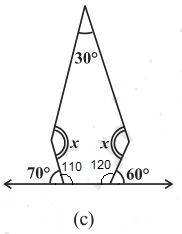Sum of angles of the pentagon = 540°
Two angles at the bottom are linear pair.
Therefore, 180° - 70° = 110°
180° - 60° = 120°
⇒ 30° + 110° + 120° + x + x = 540°
⇒ 260° + 2x = 540°
⇒ 2x = 540° - 260° = 280°
⇒ x = 280°/2 = 140°

(d) The figure is having 5 equal sides. Hence, it is a regular pentagon. Thus, its all angles are equal.
5x = 540°
⇒ x = 540°/5
⇒ x = 108°

7.(a) Sum of all angles of triangle = 180°
One side of triangle = 180°- (90° 30°) = 60°
x + 90° = 180° ⇒ x = 180° - 90° = 90°
y + 60° = 180° ⇒ y = 180° - 60° = 120°
z + 30° = 180° ⇒ z = 180° - 30° = 150°
x + y + z = 90° 120° + 150° = 360°

(b) Sum of all angles of quadrilateral = 360°
One side of quadrilateral = 360°- (60° + 80° + 120°) = 360° - 260° = 100°
x + 120° = 180° ⇒ x = 180° - 120° = 60°
y + 80° = 180° ⇒ y = 180° - 80° = 100°
z + 60° = 180° ⇒ z = 180° - 60° = 120°
w + 100° = 180° ⇒ w = 180° - 100° = 80°
x + y + z + w = 60° + 100° + 120° + 80° = 360°

Page No: 44

Exercise 3.2

1. Find x in the following figures.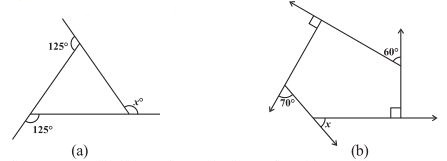(a)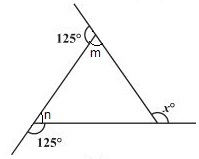125° + m = 180° ⇒ m = 180° - 125° = 55°   (Linear pair)
125° + n = 180° ⇒ n = 180° - 125° = 55°   (Linear pair)
x = m + n (exterior angle of a triangle is equal to the sum of two opposite interior two angles)
⇒ x = 55° 55° = 110°

(b)Two interior angles are right angles = 90°
70° + m = 180° ⇒ m = 180° - 70° = 110°    (Linear pair)
60° + m = 180° ⇒ m = 180° - 60° = 120°    (Linear pair)
The figure is having five sides and is a pentagon.
Thus, sum of the angles of pentagon = 540°
90° + 90° + 110° + 120° + y = 540°
⇒ 410° + y = 540° ⇒ y = 540° - 410° = 130°
x + y = 180°    (Linear pair)
⇒ x + 130° = 180°
⇒ x = 180° - 130° = 50°

2. Find the measure of each exterior angle of a regular polygon of
(i) 9 sides           (ii) 15 sides

Sum of angles a regular polygon having side n = (n-2)×180°
(i) Sum of angles a regular polygon having side 9 = (9-2)×180°
= 7×180° = 1260°
Each interior angle = 1260°/9 = 140°
Each exterior angle = 180° - 140° = 40°
Or,
Each exterior angle = Sum of exterior angles/Number of sides = 360°/9 = 40°

(i) Sum of angles a regular polygon having side 15 = (15-2)×180°
= 13×180° = 2340°
Each interior angle = 2340°/15 = 156°
Each exterior angle = 180° - 156° = 24°
Or,
Each exterior angle = Sum of exterior angles/Number of sides = 360°/15 = 24°

3. How many sides does a regular polygon have if the measure of an exterior angle is 24°?

Each exterior angle = Sum of exterior angles/Number of sides
24° = 360°/Number of sides
⇒ Number of sides = 360°/24° = 15
Thus, the regular polygon have 15 sides.

4. How many sides does a regular polygon have if each of its interior angles is 165°?

Interior angle = 165°
Exterior angle = 180° - 165° = 15°
Number of sides = Sum of exterior angles/exterior angle
⇒ Number of sides = 360°/15° = 24
Thus, the regular polygon have 24 sides.

5. (a) Is it possible to have a regular polygon with measure of each exterior angle as 22°?
(b) Can it be an interior angle of a regular polygon? Why?

(a) Exterior angle = 22°
Number of sides = Sum of exterior angles/exterior angle
⇒ Number of sides = 360°/22° = 16.36
No, we can't have a regular polygon with each exterior angle as 22° as it is not divisor of 360.

(b) Interior angle = 22°
Exterior angle = 180° - 22°= 158°
No, we can't have a regular polygon with each exterior angle as 158° as it is not divisor of 360.

6. (a) What is the minimum interior angle possible for a regular polygon? Why?
(b) What is the maximum exterior angle possible for a regular polygon?